• 图像骨架是一种图像对象结构的表示方法，在基于汉字的图像检索中起着举足轻重的作用．...通过程序实现算法分析和比较不同汉字图像骨架的提取效果，并给出针对识别不同的汉字图像应选择的最佳图像识别算法
• 1 骨架细化原理 思想： 公式: y = p0*2^0 + p1*2^1+ p2*2^2 + p3*2^3 + p4*2^4 + p5*2^5 + p6*2^6 +p7*2^7 前辈们对此作出了总结，得出每个点周围8领域的256种情况，放在一个char data的数组中，不可以...
1 骨架细化原理
思想：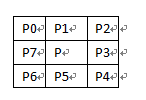公式: y = p0*2^0 + p1*2^1+ p2*2^2 + p3*2^3 + p4*2^4 + p5*2^5 + p6*2^6 +p7*2^7
前辈们对此作出了总结，得出每个点周围8领域的256种情况，放在一个char data的数组中，不可以删除用0来表示，能被删除的用1来表示。然后对图像进行处理得到二值图像<0和1>，扫描图像，根据公式得出y，依次用data[y]判断该点是否可以被删除，直到所有的点都不可以被删除为止。
算法流程图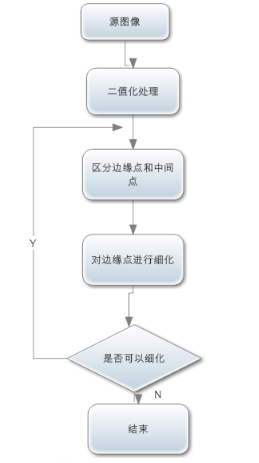原文：https://blog.csdn.net/lu597203933/article/details/14397605

2 算法实现代码
// 骨架细化.cpp : 此文件包含 "main" 函数。程序执行将在此处开始并结束。

#include "pch.h"
#include <iostream>
#include <opencv2/opencv.hpp>
#include <opencv2/core/core.hpp>
#include <iostream>
#include <vector>
#include "assert.h"

using namespace std;
using namespace cv;

Mat dst;
void Rosenfeld(Mat& src, Mat& dst)
{

if (src.type() != CV_8UC1)
{
printf("只能处理二值或灰度图像\n");
return;
}
//非原地操作时候，copy src到dst
if (dst.data != src.data)
{
src.copyTo(dst);
}

int i, j, n;
int width, height;
//之所以减1，是方便处理8邻域，防止越界
width = src.cols - 1;
height = src.rows - 1;
int step = src.step;
int  p2, p3, p4, p5, p6, p7, p8, p9;
uchar* img;
bool ifEnd;
Mat tmpimg;
int dir = { -step, step, 1, -1 };
while (1)
{
//分四个子迭代过程，分别对应北，南，东，西四个边界点的情况
ifEnd = false;
for (n = 0; n < 4; n++)
{
dst.copyTo(tmpimg);
img = tmpimg.data;
for (i = 1; i < height; i++)
{
img += step;
for (j = 1; j < width; j++)
{
uchar* p = img + j;
//如果p点是背景点或者且为方向边界点，依次为北南东西，继续循环
if (p == 0 || p[dir[n]] > 0) continue;
p2 = p[-step] > 0 ? 1 : 0;
p3 = p[-step + 1] > 0 ? 1 : 0;
p4 = p > 0 ? 1 : 0;
p5 = p[step + 1] > 0 ? 1 : 0;
p6 = p[step] > 0 ? 1 : 0;
p7 = p[step - 1] > 0 ? 1 : 0;
p8 = p[-1] > 0 ? 1 : 0;
p9 = p[-step - 1] > 0 ? 1 : 0;
//8 simple判定
int is8simple = 1;
if (p2 == 0 && p6 == 0)
{
if ((p9 == 1 || p8 == 1 || p7 == 1) && (p3 == 1 || p4 == 1 || p5 == 1))
is8simple = 0;
}
if (p4 == 0 && p8 == 0)
{
if ((p9 == 1 || p2 == 1 || p3 == 1) && (p5 == 1 || p6 == 1 || p7 == 1))
is8simple = 0;
}
if (p8 == 0 && p2 == 0)
{
if (p9 == 1 && (p3 == 1 || p4 == 1 || p5 == 1 || p6 == 1 || p7 == 1))
is8simple = 0;
}
if (p4 == 0 && p2 == 0)
{
if (p3 == 1 && (p5 == 1 || p6 == 1 || p7 == 1 || p8 == 1 || p9 == 1))
is8simple = 0;
}
if (p8 == 0 && p6 == 0)
{
if (p7 == 1 && (p3 == 9 || p2 == 1 || p3 == 1 || p4 == 1 || p5 == 1))
is8simple = 0;
}
if (p4 == 0 && p6 == 0)
{
if (p5 == 1 && (p7 == 1 || p8 == 1 || p9 == 1 || p2 == 1 || p3 == 1))
is8simple = 0;
}
adjsum = p2 + p3 + p4 + p5 + p6 + p7 + p8 + p9;
//判断是否是邻接点或孤立点,0,1分别对于那个孤立点和端点
if (adjsum != 1 && adjsum != 0 && is8simple == 1)
{
dst.at<uchar>(i, j) = 0; //满足删除条件，设置当前像素为0
ifEnd = true;
}

}
}
}
if (!ifEnd) break;
}

}

int main(int argc, char*argv[])
{

if (src.empty())
{
cout << "读取文件失败！" << std::endl;
return -1;
}
resize(src, src, Size(src.cols / 2, src.rows / 2), (0, 0), (0, 0), 3);
//将原图像转换为二值图像
threshold(src, src, 20, 255, THRESH_BINARY_INV);//THRESH_BINARY_INV和 THRESH_BINARY不同对骨架细化有影响
imshow("二值图像", src);
//图像细化
Rosenfeld(src, dst);
//显示图像
dst = dst * 255;
imshow("未细化图片", src);
imshow("细化图片", dst);
waitKey(0);
return 0;
}


效果图展示
原始图1（采用THRESH_BINARY_INV）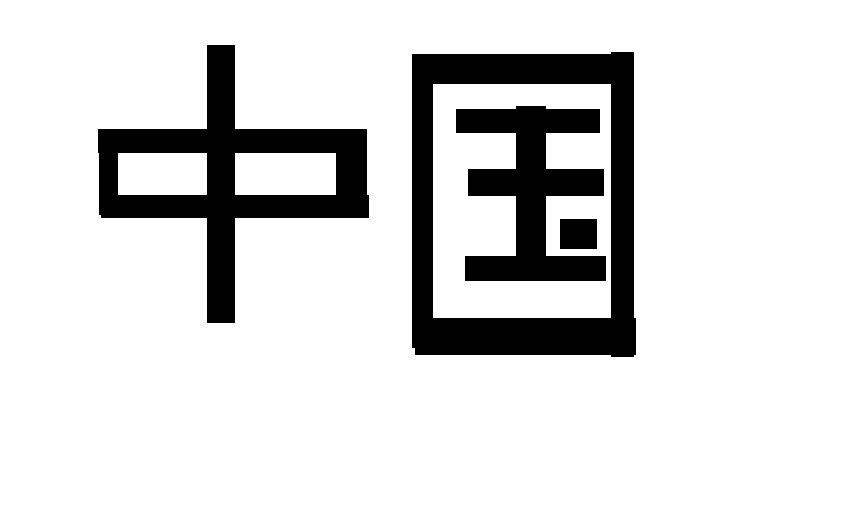效果图1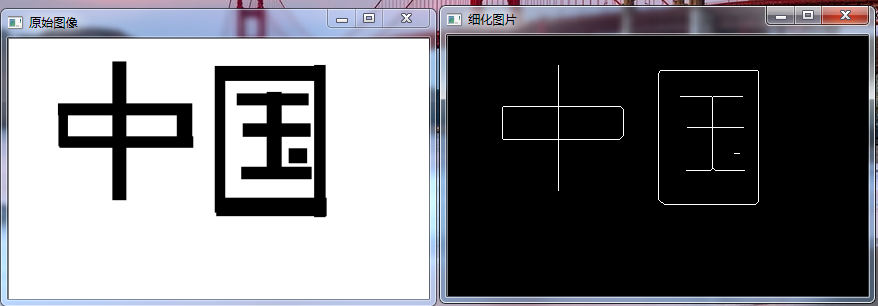原始图2（采用THRESH_BINARY）效果图2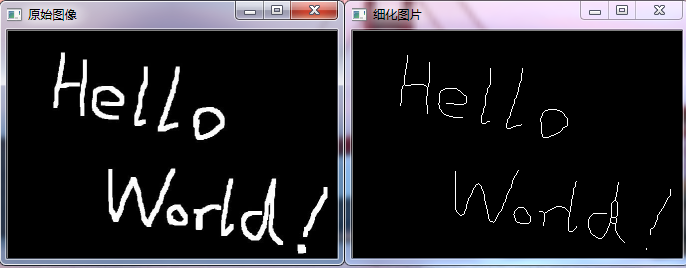注意 注意 注意！！！！！！！！！！！！
因为骨架细化算法输入的图片是二值化图片或者灰度图片  这里统一将图片定义为二值化图片后在输入：
如果前景是黑色背景是白色（即字符是黑色背景是白色） 使用THRESH_BINARY_INV（取反操作）二值化后（即字符是白色背景是黑色）
之后就可以将图片输入算法中进行骨架细化。
如果前景是白色背景是黑色（即字符是白色背景是黑色） 使用THRESH_BINARY二值化后（即字符是白色背景是黑色）
之后就可以将图片输入算法中进行骨架细化。
概括一下这个算法要求的设置是：待细化的图片前景是白色即用1表示；背景是黑色的用0表示
即最后输入的照片一定是前景（要细化的细节）是白色背景是黑色（图片底色）
也可以自己更改参数修改！！！！！！！！！！！！！

展开全文• 博士论文《光干涉图像的滤波和骨架化算法研究.kdh》 摘 要 随着激光技术和计算机技术的快速发展，现代光学测量技术已经广泛应用于 微位移测量、波面复原及物体表面形貌重构等科研和和工程领域。与传统的光学 测量...
• 骨架提取与分水岭算法也属于形态学处理范畴，都放在morphology子模块内。 1、骨架提取 骨架提取，也叫二值图像细化。这种算法能将一个连通区域细化成一个像素的宽度，用于特征提取和目标拓扑表示。 morphology子模块...
• 分析串行骨架细化算法各步骤中包含的可并行操作，对其进行并行设计，提出的算法通过OpenMP多线程技术实现，并采用不同大小的三维CT血管图像进行分析和测试。根据测试结果，改进后的算法获取到的骨架准确可靠，...
• 圖像的細是模式識別中很重要的一個技術，指的是將原本"臃腫"的像素簡為單像素相連接的二值圖像（即類似骨架的概念），細的好壞直接影響到後面識別匹配的效率。 摘自某文章的話，細就是經過一層層的剝離，...
http://www.cnblogs.com/blue-lg/archive/2012/03/08/2383955.html

圖像的細化是模式識別中很重要的一個技術，指的是將原本"臃腫"的像素簡化為單像素相連接的二值圖像（即類似骨架的概念），細化的好壞直接影響到後面識別匹配的效率。
摘自某文章的話，細化就是經過一層層的剝離，從原來的圖中去掉一些點，但仍要保持原來的形狀，直到得到圖像的骨架。骨架，可以理解為圖象的中軸，例如一個長方形的骨架是它的長方向上的中軸線；正方形的骨架是它的中心點；圓的骨架是它的圓心，直線的骨架是它自身，孤立點的骨架也是自身。

下面先介紹經典的Zhang並行快速細化算法：
設p1點的八鄰域為：
【    p9    p2     p3
p8    p1     p4
p7    p6     p5 】
（其中p1為白點，如果以下四個條件同時滿足，則刪除p1，即令p1=0）
其中迭代分為兩個子過程：
過程1 細化刪除條件為：        （1）、2 < =N(p1) <= 6，   N(x)為x的8鄰域中黑點的數目
（2）、A(p1)=1,    A(x)指的是將p2-p8之間按序前後分別為0、1的對數（背景色：0）
（3）、p2*p4*p6=0
（4）、p4*p6*p8=0
如果同時滿足以上四個條件則該點可以刪除（賦值為0）。
過程2 細化刪除條件為：        （1）、2 < =N(p1) <= 6，   N(x)為x的8鄰域中黑點的數目
（2）、A(p1)=1,    A(x)指的是將p2-p8之間按序前後分別為0、1的對數（背景色：0）
（3）、p2*p4*p8=0
（4）、p2*p6*p8=0
如果同時滿足以上四個條件則該點可以刪除。
代碼如下：
A.m1 function n=A(temp,i,j)2 %0->1的數目3 shuzu=[temp(i,j),temp(i-1,j),temp(i-1,j+1),temp(i,j+1),temp(i+1,j+1),temp(i+1,j),temp(i+1,j-1),temp(i,j-1),temp(i-1,j-1)];4 n=0;5 for i=2:86     if shuzu(i)==0&&shuzu(i+1)==17         n=n+1;8     end9 end主函數代碼：1 test=input('Please input a digits image:','s'); %輸入圖像 2 x=imread(test); 3 if ~isbw(x) 4     '請確保輸入圖像為二值化圖像！'; 5 else  6     [height,width]=size(x); 7     mark=1; 8 %     temp=zeros(height+2,width+2); 9 %     temp(2:height+1,2:width+1)=x(:,:);10     temp=x;11     imshow(temp);12    while mark==113     mark=0;14         15         for i=2:height-116             for j=2:width-117                 condition=0;18              %判斷P(r,c)是否為可細化像素19                if temp(i,j)==120                  n=0;21                  for ii=-1:122                      for jj=-1:123                          n=n+temp(i+ii,j+jj);24                      end25                  end26              if (n>=3 && n<=7)27                  condition=condition+1;28              end 29              if A(temp,i,j)==130                  condition=condition+1;31              end32              if temp(i-1,j)*temp(i,j+1)*temp(i+1,j)==033                 condition=condition+1;34             end35             if temp(i,j+1)*temp(i+1,j)*temp(i,j-1)==036                 condition=condition+1;37             end38           if condition==4           39                mark=1;40                temp(i,j)=0;41           end42                end43             end44         end45         figure;imshow(temp);46         47         48         for i=2:height-149             for j=2:width-150                 condition=0;51              %判斷P(r,c)是否為可細化像素52                if temp(i,j)==153                  n=0;54                  for ii=-1:155                      for jj=-1:156                          n=n+temp(i+ii,j+jj);57                      end58                  end59              if (n>=3 && n<=7)60                  condition=condition+1;61              end 62              if A(temp,i,j)==163                  condition=condition+1;64              end65              if temp(i-1,j)*temp(i,j+1)*temp(i,j-1)==066                 condition=condition+1;67             end68             if temp(i,j-1)*temp(i+1,j)*temp(i,j-1)==069                 condition=condition+1;70             end71           if condition==4           72                mark=1;73                temp(i,j)=0;74           end75                end76             end77         end78         figure;imshow(temp);79     end  80 end結果：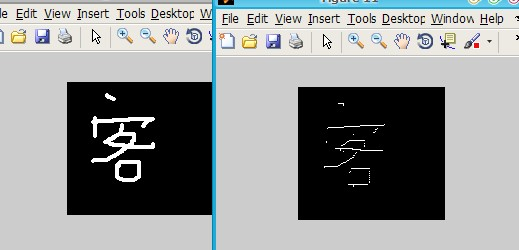展开全文input function image c
• ## 骨架提取算法应用

千次阅读 2020-01-16 10:22:11
根据个人理解，骨架提取（顾名思义）就是根据各个连通区域，将其抽离出与其轮廓近似的单像素表示形态。以便于直观观察、图像的后继处理。因此可以将其视为图像处理中的预处理，其操作是基于二值图。为了更好的提取...
1、引言 根据个人理解，骨架提取（顾名思义）就是根据各个连通区域，将其抽离出与其轮廓近似的单像素表示形态。以便于直观观察、图像的后继处理。因此可以将其视为图像处理中的预处理，其操作是基于二值图。为了更好的提取图像骨架，必要时需要对图像进行相应的预处理（比如去噪、滤波、形态学变换等）。 我的应用主要集中在对一些包含线条型的零件检测，除此之外，骨架提取的应用特别广泛，比如文字的检测/识别、道路观测等。
2、原理 Zhang和Suen提出了一种带有模板匹配的并行细化算法，生成一个像素宽的骨架，不仅保持图像的连通性，并且产生更薄的结果，保持快速的处理速度。 Zhang-Suen细化算法通常是一个迭代算法，整个迭代过程分为两步：
第一步：循环所有前景像素点，对符合如下条件的像素点标记为删除： 1)2<=N(P1)<=6 2)S(P1)=1 3)P2P4P6=0 4)P4P6P8=0 其中N(P1)表示跟P1相邻的8个像素点中，为前景像素点的个数，S(P1)表示从P2-P9-P2像素中出现0-1的累积次数，其中0表示背景，1表示前景，完整的P1-P9的像素位置分布如表1：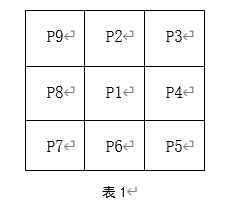第二步： 1)2<=N(P1)<=6 2)S(P1)=1 3)P2P4P8=0 4)P2P6P8=0 循环以上两个步骤，直到两步中没有像素被标记为删除为止，输出的结果即为二值图像细化后的骨架。
3、案例核心代码
//Zhang-Sun细化算法
void SkeletonExtraction()
{
//原图像名称
string Img_name = "TEST.png";
//载入源图像
Mat src = Src.clone();
//灰度化
cvtColor(src, src, COLOR_RGB2GRAY);
//Otsu求阈值
int thre = Otsu(src);
Mat Img;
//二值化
threshold(src, Img, thre, 255, THRESH_BINARY_INV);
namedWindow("原始二值化图像", 0);
imshow("原始二值化图像", Img);

Mat srcImg = Img.clone();
/****************骨架提取算法：Zhang-Suen法*****检测焊条数量************************/
vector<Point> deleteList;
int neighbourhood;
int row = srcImg.rows;
int col = srcImg.cols;
bool inOddIterations = true;
while (true) {
for (int j = 1; j < (row - 1); j++) {
uchar* data_last = srcImg.ptr<uchar>(j - 1);
uchar* data = srcImg.ptr<uchar>(j);
uchar* data_next = srcImg.ptr<uchar>(j + 1);
for (int i = 1; i < (col - 1); i++) {
if (data[i] == 255) {
int whitePointCount = 0;
neighbourhood = 1;
//判断中心点8邻域的像素特征
if (data_last[i] == 255) neighbourhood = 1;
else  neighbourhood = 0;
if (data_last[i + 1] == 255) neighbourhood = 1;
else  neighbourhood = 0;
if (data[i + 1] == 255) neighbourhood = 1;
else  neighbourhood = 0;
if (data_next[i + 1] == 255) neighbourhood = 1;
else  neighbourhood = 0;
if (data_next[i] == 255) neighbourhood = 1;
else  neighbourhood = 0;
if (data_next[i - 1] == 255) neighbourhood = 1;
else  neighbourhood = 0;
if (data[i - 1] == 255) neighbourhood = 1;
else  neighbourhood = 0;
if (data_last[i - 1] == 255) neighbourhood = 1;
else  neighbourhood = 0;
for (int k = 1; k < 9; k++) {
//二进制值为1的个数
whitePointCount += neighbourhood[k];
}
//条件①2<=B(p1)<=6
if ((whitePointCount >= 2) && (whitePointCount <= 6)) {
int ap = 0;
//条件②A(p1)值
if ((neighbourhood == 0) && (neighbourhood == 1)) ap++;
if ((neighbourhood == 0) && (neighbourhood == 1)) ap++;
if ((neighbourhood == 0) && (neighbourhood == 1)) ap++;
if ((neighbourhood == 0) && (neighbourhood == 1)) ap++;
if ((neighbourhood == 0) && (neighbourhood == 1)) ap++;
if ((neighbourhood == 0) && (neighbourhood == 1)) ap++;
if ((neighbourhood == 0) && (neighbourhood == 1)) ap++;
if ((neighbourhood == 0) && (neighbourhood == 1)) ap++;
if (ap == 1) {
if (inOddIterations && (neighbourhood * neighbourhood * neighbourhood == 0)
&& (neighbourhood * neighbourhood * neighbourhood == 0)) {
deleteList.push_back(Point(i, j));
}
else if (!inOddIterations && (neighbourhood * neighbourhood * neighbourhood == 0)
&& (neighbourhood * neighbourhood * neighbourhood == 0)) {
deleteList.push_back(Point(i, j));
}
}
}
}
}
}
if (deleteList.size() == 0)
break;
for (size_t i = 0; i < deleteList.size(); i++) {
Point tem;
tem = deleteList[i];
uchar* data = srcImg.ptr<uchar>(tem.y);
data[tem.x] = 0;
}
deleteList.clear();

inOddIterations = !inOddIterations;
}
namedWindow("骨架提取", 0);
imshow("骨架提取", srcImg);
}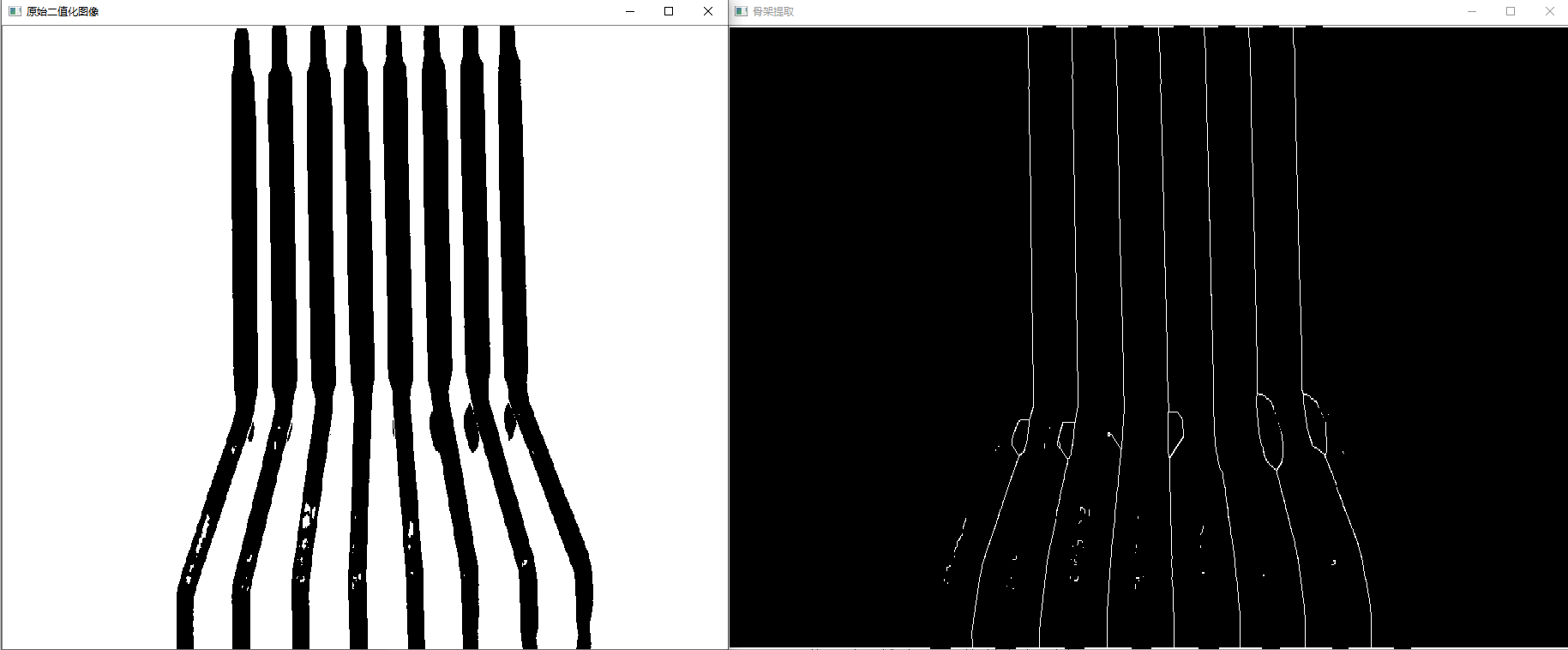展开全文• 图像骨架提取，实际上就是提取目标在图像上的中心像素轮廓。...关于骨架提取，现存的算法有一千种以上（论文中谈到，There are more than one thousand algorithms that have been published ...
图像骨架提取，实际上就是提取目标在图像上的中心像素轮廓。说白了就是以目标中心为准，对目标进行细化，一般细化后的目标都是单层像素宽度。比如输入图像是这样：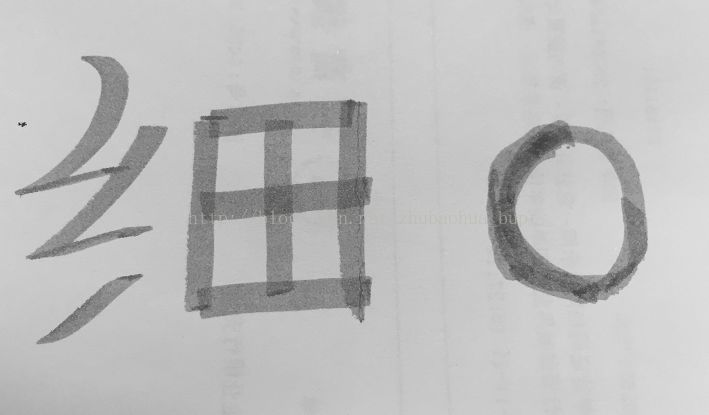输出骨架图像(红色)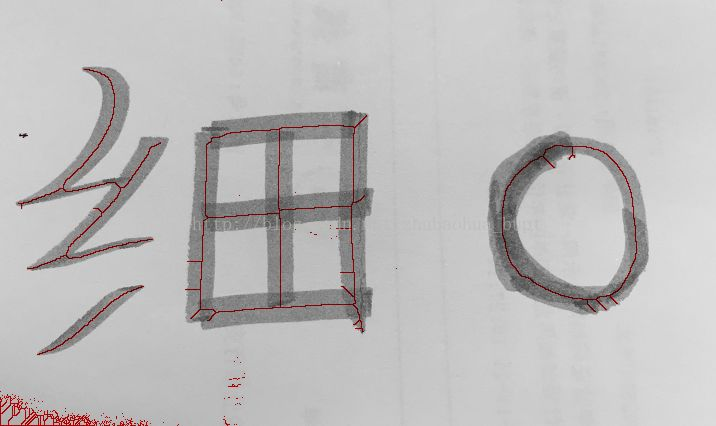关于骨架提取，现存的算法有一千种以上（论文中谈到，There are more than one thousand algorithms that have been published on this topic）。论文中提到，所有的骨架提取算法无外乎分为迭代和非迭代两大类。在迭代算法中，又分为并行迭代和顺序迭代两种。

本文分别介绍两种提取算法，它们分别来源于论文(K3M顺序迭代)、(Zhang-Suenalgorithm,并行迭代)。

这两种方法都是对二值图像操作的，思想都是从目标外围往目标中心，利用以待检测像素为中心3*3像素窗口的特征，对目标不断腐蚀细化，直至腐蚀到不能再腐蚀（单层像素宽度），就得到了图像的骨架。下面详细介绍一下两种骨架提取算法。

一 K3M

该算法的思想是，提取目标外围轮廓，然后利用轮廓腐蚀目标图像边界（这是一次迭代过程），直至腐蚀到不能再腐蚀。

算法分为两块
第一块，不断腐蚀，提取出伪骨架(部分区域有两层像素宽度，但已经很接近真实骨架)。
第二块，从伪骨架中提取真实骨架。

第一块：提取出伪骨架
每次迭代共有6步。不断迭代，直至某次迭代的过程，目标边界中没有新的像素被腐蚀，那么此时，剩余的目标图像像素就很接近于真实骨架(称之为伪骨架)。

<1>提取最新目标轮廓(初始为原目标轮廓)并记录这些轮廓点。

<2>依次检测这些轮廓点的8像素邻域，是否只含有3连通像素，如果有，把此点从轮廓点删除，并在目标图像中删除（腐蚀掉）对应点。

<3>依次检测<2>中剩余轮廓点的8像素邻域，是否只含有3 or 4连通像素，如果有，把此点从轮廓点删除，并在目标图像中删除（腐蚀掉）对应点。

<4>依次检测<3>中剩余轮廓点的8像素邻域，是否只含有3 or 4 or 5连通像素，如果有，把此点从轮廓点删除，并在目标图像中删除（腐蚀掉）对应点。

<5>依次检测<4>中剩余轮廓点的8像素邻域，是否只含有3 or 4 or 5 or 6连通像素，如果有，把此点从轮廓点删除，并在目标图像中删除（腐蚀掉）对应点。

<6>依次检测<5>中剩余轮廓点的8像素邻域，是否只含有3 or 4 or 5 or 6 or 7连通像素，如果有，把此点从轮廓点删除，并在目标图像中删除（腐蚀掉）对应点。

这是一次迭代过程的最后一步，如果在这步骤中仍有像素被腐蚀，说明除了真实骨架还有”肉”，仍需继续腐蚀边界。这一步骤是算法迭代的终止条件。

第二块 从伪骨架中提取真实骨架
算法第一块得到的伪骨架有部分区域是两个像素宽度，而目标骨架是单层像素宽度。所以，通过下面这一步骤提取最终骨架：

依次检测目标图像中，伪骨架的8像素邻域，是否只含有2 or 3 or 4 or 5 or 6 or 7连通像素，如果有，把此点从伪骨架中删除(腐蚀)，就得了最终的骨架。

该论文对像素p的8邻域进行了权重编码：对判断像素点p周围8邻域的最大连通区域，是否只含有n（2<n<7）连通像素，进行加速。如下图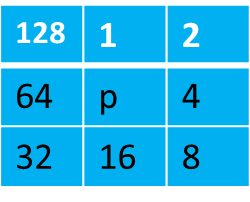比如判断点p的8邻域是否只含有2连通的区域，可以这样实现：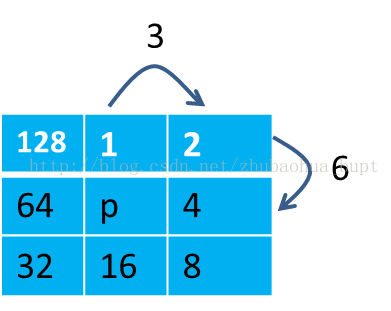把相邻2连通像素两两相加，会得到一个这样的集合c2{3,6,12,24,48,96,192,129},
由于操作的是二值图像，在判断p的8邻域的最大连通域是否只含有2连通域时，只需要对p的8邻域进行加权相加得到一个值，记作p2，然后去c2里查询，如果c2里有p2，则点p周围的8邻域只含有2连通的区域。比如：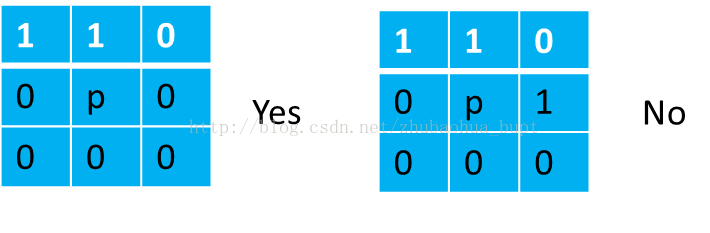这样的加权方式，使得每个可能出现的n（2<n<7）连通邻域像素区域都对应一个不同的值。

原文中查询集合：
A1=3连通,{}
A2=3or4连通,
A3=3 or 4 or 5连通,
A4=3 or 4 or 5 or 6连通,
A5=3 or 4 or 5 or 6 or 7连通
A0=2 or3 or 4 or 5 or 6 or 7连通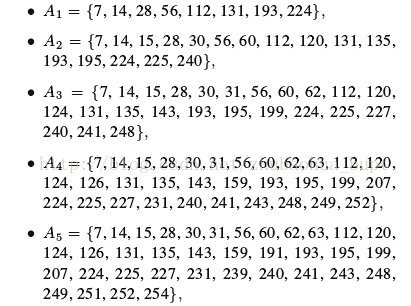需要注意的是，每次迭代中的每一小步顺序不能变，否则会在腐蚀拐点的时候出现漏网之鱼，随着迭代的次数增加，效果就会和真实的骨架偏差越来越大。

二 Zhang-Suen algorithm

这个算法是论文提出的，该算法每一次的迭代步骤是对符合特定条件的目标像素，进行腐蚀，效果就是目标变得越来越细。不断的迭代，直到在上一次腐蚀后的目标在本轮操作中，没有新的像素点被腐蚀，算法结束。

下面说一下此算法的四个条件：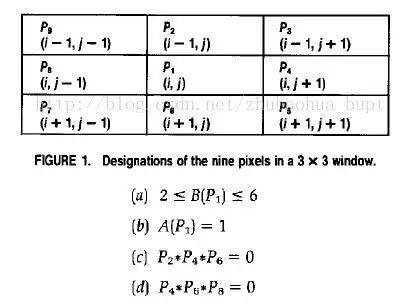条件(a)：中心像素P1周围的目标像素（二值中的1）的个数之和在2和6之间。
条件(b): 8邻域像素中，按顺时针方向，相邻两个像素出现0->1的次数。
比如，下面这个例子,A(P1)=2。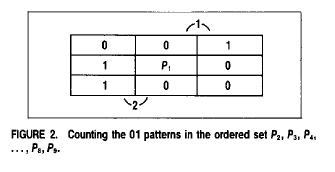条件(c)(d)有两种模式，一种上面提到过，下面是另一种。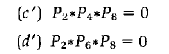Zhang-Suen thinning steps:
While points are deleted do
For all pixels p(i,j) do
if (a)2 ≤ B(P1) ≤ 6 (b) A(P1) = 1 (c) Apply oneof the following: 1. P2 x P4 x P6 = 0 in odd iterations 2. P2 x P4 x P8 = 0 in even iterations (d) Apply oneof the following: 1. P4 x P6 x P8 = 0 in odd iterations 2. P2 x P6 x P8 = 0 in even iteration
then
Deletepixel p(i,j)
endif
end for
end while

算法代码实现：https://github.com/ExtremeMart/SkeletonExtraction

参考论文
K3M: A UNIVERSAL ALGORITHM FOR IMAGE SKELETONIZATION AND A REVIEW OF THINNING TECHNIQUES
A fast parallel algorithm for thinning digital patterns
展开全文• 记录一下图像骨架提取算法，转载至 两种图像骨架提取算法的研究(1)原理部分基于OpenCV的实现代码如下，代码参考 opencv骨架提取/图像细化void Utilities::thinImage(Mat &amp; srcImg) { vector&lt;Point&...OpenCV
• 视觉组学习内容：Zhang-Suen骨架提取算法欢迎使用Markdown编辑器任务要求如何插入一段漂亮的代码片生成一个适合你的列表创建一个表格设定内容居中、居左、居右SmartyPants创建一个自定义列表如何创建一个注脚注释也...opencv Ubuntu
• 针对单目网像序列中跟踪人体运动时，人体骨架模型难以自动提取的问题。提出一种在人体正面运动的条件下自动提取人体骨架模型的方法。...实验结果显示，该算法能够较精确地定位关节点位置，自动提取出人体尺度骨架模型。
• 基于地面三维激光扫描仪获取树木的点云数据,提出了一种通过细化点云...实验结果表明,所提算法生成的两棵树木骨架形态与树木原始点云所表现的形态结构相对一致,并且具有较好的运算效率,该算法具有一定的可行性和有效性。
• 骨骼追踪 ... 尽管可以使用轮廓查找来进一步跟踪结果，但是它们通常会给出封闭的轮廓，而不是单个笔触，并且由于骨架化过程的不完善而导致笔触宽度容易出现细微变化。 在此演示中，我们提出了一种基于可并
• 将改进后的算法应用于脱机手写体数字骨架化，实验证明，改进的算法克服了上述缺点，能更好地找到数据的拓扑结构，在时间复杂度、连通性、参数等方面均优于其他改进算法。改进算法适于具有“连通性”的数据。
• 基于 OpenCV 的 C++ 代码， Visual Studio 2017 工程，算法主要功能：提取图像中形状的骨架主干部分并细化
• 骨架提取与分水岭算法也属于形态学处理范畴，都放在morphology子模块内。 1、骨架提取 骨架提取，也叫二值图像细化。这种算法能将一个连通区域细化成一个像素的宽度，用于特征提取和目标拓扑表示。 morphology子...
• ## 分水岭算法及案例

万次阅读 多人点赞 2017-11-29 13:04:32
分水岭算法Watershed Algorithm(分水岭算法)，顾名思义，就是根据分水岭的构成来考虑图像的分割。现实中我们可以或者说可以想象有山有湖的景象，那么那一定是水绕 山，山围水的情形。当然在需要的时候，要人工构筑...图像处理 图像分割
• 这种算法能将一个连通区域细化成一个像素的宽度，用于特征提取和目标拓扑表示。 morphology子模块提供了两个函数用于骨架提取，分别是Skeletonize（）函数和medial_axis（）函数。我们先来看Skeletonize（）函数。 ...
• 一、为什么需要骨架提取 简单来说就是用于细化栅格，便于栅格数据转换为矢量数据 栅格格式向矢量格式转换是提取相同编号的栅格集合表示的边界，栅格点转换成矢量点，很简单，在坐标系确定的情况下通过解析式可以...
• 关注2-D骨架提取问题,将其算法分为骨架提取的对称轴分析方法、细化方法以及形状分解方法 3大类,分别给出了这3类方法的基本思想和发展脉络,希望通过这样的工作为模式识别、可视和医学图像处理等相关领域的同行提供...
• MATLAB有多种对二值图像进行骨架化的方法，以下图为例： I = imread('threads.jpg'); I = imbinarize(I); % 需要首先对图像进行二值化 第一种方法： bw1 = bomorph(I,'thin',Inf); figure imshow(bw1) 第二种方法...
• 形态学骨架化及重建 形态学骨架化及重建 1. 前言 2. 形态学骨架化及重建描述 3. 代码实现 1. 前言 数学形态学（Mathematical Morphology）是分析几何形状和结构的数学方法，是建立在集合代数基础上，用集合...图像形态学 图像细化 计算机视觉 人工智能
• cv::imshow("二值图像", binaryImage * 255); thin(binaryImage); cv::imshow("骨架图像", binaryImage*255); cv::cvtColor(raw, raw, CV_GRAY2BGR); for(int row=0;row;row++) for (int col = 0; col ; ...
• 昨天不是说同学问我怎么绘制出轮廓的中心线。然后我上网查了一下其实这个有专门的算法叫做细化...图像细化(Image Thinning)，一般指二值图像的骨架化(Image Skeletonization)的一种操作运算。切记：前提条件一定是二...骨骼提取 opencv
• ## 形态学骨架提取

千次阅读 2018-11-23 15:00:10
下面我来介绍一下关于形态学中的骨架提取，并在MATLAB中实现： 1、打开MATLAB软件，在其主界面的编辑器中写入下列程序： I=imread('G:\MATLAB\bm.bmp'); %读取当前路径下的图片 subplot(2,2,1),imshow(I); title...MATLAB实现
• 细化提取血管中心线，itk读取和输出mhd图像，最终生成血管中心线树结构，实现算法K. Palágyi, E. Balogh, A. Kuba, C. Halmai, B. Erdőhelyi, E. Sorantin, K. Hausegger, A sequential 3D thinning algorithm and...
• //根据上述细化算法，在OpenCV中采用3×3结构元素的细化代码如下： //函数名称：Mat thinning() //基本功能：对二值图像进行细化运算 //参数说明：待细化二值图像，该图像中背景色为0，前景色(目标)为255 //返回值：...opencv 计算机视觉
• 植物建模是计算机图形学研究热点之一，提出了一种园林植物数字建模算法。该算法通过园林植物的数字表示方法以及四个主要步骤实现仿真效果。第一步初始空间网格结构以及植物的轮廓结构；第二步通过生长范围计算......